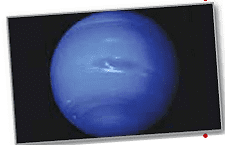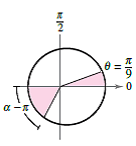Create an Account

Home / Questions / Planetary Motion In Exercise 63, the polar equation for the elliptical orbit of Neptune wa...

Planetary Motion In Exercise 63, the polar equation for the elliptical orbit of Neptune was found. Use the equation and a computer algebra system to perform each of the following. Chapter 10.6, Probl

Planetary Motion In Exercise 63, the polar equation for the elliptical orbit of Neptune was found. Use the equation and a computer algebra system to perform each of the following.(a) Approximate the area swept out by a ray from then sun to the planet asincreases from 0 to. Use this result to determine the number of years required for the planet to move through this arc when the period of one revolution around the sun is 165 years.

(b) By trial and error, approximate the anglesuch that the area swept out by a ray from the sun to the planet asincreases fromtoequals the area found in part (a) (see figure). Does the ray sweep through a larger or smaller angle than in part (a) to generate the same area? Why is this the case?(c) Approximate the distances the planet traveled in parts (a) and (b). Use these distances to approximate the average number of kilometers per year the planet traveled in the two cases.

Jul 31 2021 View more View LessSubscribe To Get Solution